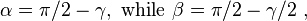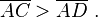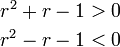# TRIANGLE INEQUALITY

In mathematics, the triangle inequality states that for any triangle, the sum of the lengths of any two sides must be greater than the length of the remaining side.

In Euclidean geometry and some other geometries the triangle inequality is a theorem about distances. In Euclidean geometry, for right triangles it is a consequence of Pythagoras’ theorem, and for general triangles a consequence of the law of cosines, although it may be proven without these theorems. The inequality can be viewed intuitively in either R2 or R3. The figure at the right shows three examples beginning with clear inequality (top) and approaching equality (bottom). In the Euclidean case, equality occurs only if the triangle has a 180° angle and two 0° angles, making the three vertices collinear, as shown in the bottom example. Thus, in Euclidean geometry, the shortest distance between two points is a straight line.

In spherical geometry, the shortest distance between two points is an arc of a great circle, but the triangle inequality holds provided the restriction is made that the distance between two points on a sphere is the length of a minor spherical line segment (that is, one with central angle in [0, π]) with those endpoints.

The triangle inequality is a defining property of norms and measures of distance. This property must be established as a theorem for any function proposed for such purposes for each particular space: for example, spaces such as the real numbers, Euclidean spaces, the Lp spaces (p ≥ 1), and inner product spaces.

## Euclidean geometryEuclid’s construction for proof of the triangle inequality for plane geometry.

Euclid proved the triangle inequality for distances in plane geometry using the construction in the figure. Beginning with triangle ABC, an isosceles triangle is constructed with one side taken as BC and the other equal leg BD along the extension of side AB. It then is argued that angle β > α, so side AD > AC. But AD = AB + BD = AB + BC so the sum of sides AB + BC > AC. This proof appears in Euclid’s Elements, Book 1, Proposition 20.

### Right triangleIsosceles triangle with equal sides AB = AC divided into two right triangles by an altitude drawn from one of the two base angles.

A specialization of this argument to right triangles is:

In a right triangle, the hypotenuse is greater than either of the two sides, and less than their sum.

The second part of this theorem already is established above for any side of any triangle. The first part is established using the lower figure. In the figure, consider the right triangle ADC. An isosceles triangle ABC is constructed with equal sides AB = AC. From the triangle postulate, the angles in the right triangle ADC satisfy:Likewise, in the isosceles triangle ABC, the angles satisfy:Therefore,and so, in particular,

That means side AD opposite angle α is shorter than side AB opposite the larger angle β. But AB = AC. Hence:\overline{AD} \ . ” />

A similar construction shows AC > DC, establishing the theorem.

An alternative proof (also based upon the triangle postulate) proceeds by considering three positions for point B: (i) as depicted (which is to be proven), or (ii) B coincident with D (which would mean the isosceles triangle had two right angles as base angles plus the vertex angle γ, which would violate the triangle postulate), or lastly, (iii) B interior to the right triangle between points A and D (in which case angle ABC is an exterior angle of a right triangle BDC and therefore larger than π/2, meaning the other base angle of the isosceles triangle also is greater than π/2 and their sum exceeds π in violation of the triangle postulate).

This theorem establishing inequalities is sharpened by Pythagoras’ theorem to the equality that the square of the length of the hypotenuse equals the sum of the squares of the other two sides.

### Relationship with shortest pathsThe arc length of a curve is defined as the least upper bound of the lengths of polygonal approximations.

The triangle inequality can be used to prove that the shortest curve between two points in Euclidean geometry is a straight line. First, the triangle inequality can be extended by mathematical induction to arbitrary polygonal paths, showing that the total length of such a path is no less than the length of the straight line between its endpoints. Thus no polygonal path between two points is shorter than the line between them.

The result for polygonal paths implies that no curve can have an arc length less than the distance between its endpoints. By definition, the arc length of a curve is the least upper bound of the lengths of all polygonal approximations of the curve. The result for polygonal paths shows that the straight line between the endpoints is shortest of all the polygonal approximations. Because the arc length of the curve is greater than or equal to the length of every polygonal approximation, the curve itself cannot be shorter than the straight line path.

###  Some practical examples of the use of the inequality

Consider a triangle whose sides are in an arithmetic progression and let the sides be a, a + d, a + 2d. Then the triangle inequality requires that :-

To satisfy all these inequalities requires :-0 \,” />   and   <img src=”http://upload.wikimedia.org/math/f/d/5/fd5d80163c6551c8e728e8e616c627bb.png&#8221; alt=” -\frac{a}{3}<d

When d is chosen such that d = a/3, it generates a right triangle that is always similar to the Pythagorean triple with sides 3, 4, 5.

Now consider a triangle whose sides are in a geometric progression and let the sides be a, ar, ar2. Then the triangle inequality requires that :-

The first inequality requires a > 0, consequently it can be divided through and eliminated. With a > 0, the middle inequality only requires r > 0. This now leaves the first and third inequalities needing to satisfy :-0 \\
r^2-r-1 & {}

The first of these quadratic inequalities requires r to range in the region beyond the value of the positive root of the quadratic equation
r2 + r − 1 = 0, i.e. r > φ − 1 where φ is the golden ratio. The second quadratic inequality requires r to range between 0 and the positive root of the quadratic equation r2 − r − 1 = 0, i.e. 0 < r < φ. The combined requirements result in r being confined to the range

When r the common ratio is chosen such that r = √φ it generates a right triangle that is always similar to the Kepler triangle.

RELATED POST ::